# Pythagoras Theorem

Go back to  'Triangles-Quadrilaterals'

Now we will discuss an interesting proof of the Pythagoras Theorem using the concepts we have covered in this chapter. Consider $$\Delta ABC$$ which is right-angled at A: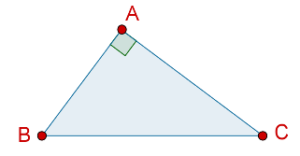We need to show that AB2 + AC2 = BC2. We begin by drawing squares on each of the three sides of the triangle: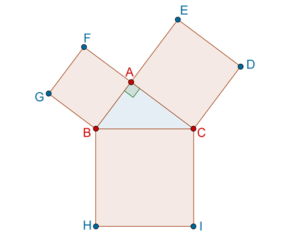Thus, effectively what we need to show is that:

area(Square ABGF) + area(Square CAED) = area(Square BCIH)

Do the following construction: join G to C, A to H, and draw a line through A perpendicular to BC, as show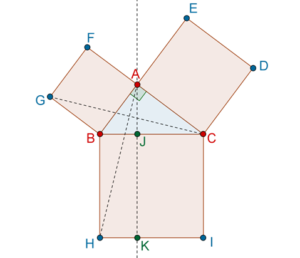We note that $$\Delta GBC$$ ≡ $$\Delta ABH$$, because:

1.  GB = AB (sides of the same square)
2.  BC = BH (sides of the same square)
3.  $$\angle GBC$$ = 900 + $$\angle ABC$$ = $$\angle ABH$$

Thus, the SAS congruence criterion applies. Since the two triangles are congruent, they will have the same area. Now comes the important part. Note that following:

1. $$\Delta GBC$$ and square GBAF are on the same base GB and between the same parallels, which means that area(GBAF) = 2 × area($$\Delta GBC$$).

2. $$\Delta ABH$$ and rectangle BHKJ are on the same base BH and between the same parallels, which gives area(BHJK) = 2 × area($$\Delta ABH$$).

From these two observations, we conclude that area(ABGF) = area(BHKJ). This is highlighted in the figure below: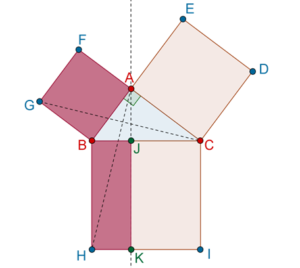Similarly, we can conclude that area(CAED) = area(CIKJ), as highlighted below: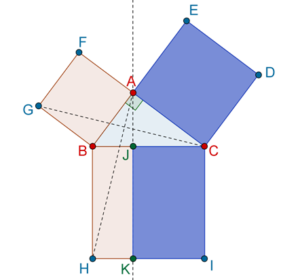Clearly, if we add the areas of two smaller squares, we get the area of the larger square:

area(Square ABGF) + area(Square CAED) = area(Square BCIH)